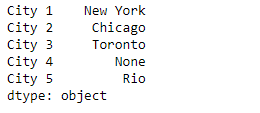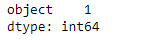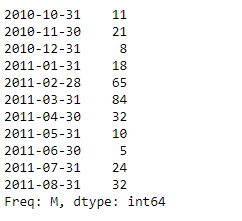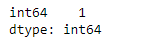# Python | Pandas Series.get_dtype_counts()

• Last Updated : 13 Feb, 2019

Pandas series is a One-dimensional ndarray with axis labels. The labels need not be unique but must be a hashable type. The object supports both integer- and label-based indexing and provides a host of methods for performing operations involving the index.

Pandas` Series.get_dtype_counts()` function return the counts of unique dtypes in this object.

Syntax: Series.get_values()

Parameter : None

Returns : dtype : Series

Example #1: Use `Series.get_dtype_counts()` function to return the count of unique dtype in the given series object.

 `# importing pandas as pd ` `import` `pandas as pd ` ` `  `# Creating the Series ` `sr ``=` `pd.Series([``'New York'``, ``'Chicago'``, ``'Toronto'``, ``None``, ``'Rio'``]) ` ` `  `# Create the Index ` `index_ ``=` `[``'City 1'``, ``'City 2'``, ``'City 3'``, ``'City 4'``, ``'City 5'``]  ` ` `  `# set the index ` `sr.index ``=` `index_ ` ` `  `# Print the series ` `print``(sr) `

Output :Now we will use `Series.get_dtype_counts()` function to return the count of unique dytpe in the given series object.

 `# return the count of dtypes ` `result ``=` `sr.get_dtype_counts() ` ` `  `# Print the result ` `print``(result) `

Output :As we can see in the output, the `Series.get_dtype_counts()` function has returned the count of dtype in the given series object. It has returned object.

Example #2 : Use `Series.get_dtype_counts()` function to return the count of unique dtype in the given series object.

 `# importing pandas as pd ` `import` `pandas as pd ` ` `  `# Creating the Series ` `sr ``=` `pd.Series([``11``, ``21``, ``8``, ``18``, ``65``, ``84``, ``32``, ``10``, ``5``, ``24``, ``32``]) ` ` `  `# Create the Index ` `index_ ``=` `pd.date_range(``'2010-10-09'``, periods ``=` `11``, freq ``=``'M'``) ` ` `  `# set the index ` `sr.index ``=` `index_ ` ` `  `# Print the series ` `print``(sr) `

Output :Now we will use `Series.get_dtype_counts()` function to return the count of unique dytpe in the given series object.

 `# return the count of dtypes ` `result ``=` `sr.get_dtype_counts() ` ` `  `# Print the result ` `print``(result) `

Output :As we can see in the output, the `Series.get_dtype_counts()` function has returned the count of dtype in the given series object. It has returned int64.

My Personal Notes arrow_drop_up
Recommended Articles
Page :